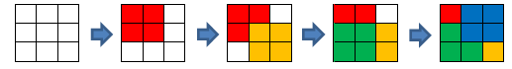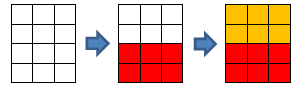CF359A Table

• 23通过
• 45提交
• 题目来源
• 评测方式 RemoteJudge
• 标签
• 难度 入门难度
• 时空限制 1000ms / 256MB
• 提示：收藏到任务计划后，可在首页查看。

题意翻译

Simon 有一个 n × m 的矩形，由（1，1）到（n，m）从左到右从上到下标号。第 x 行第 y 列的位置可以编成 (x，y)，而四个角的坐标是 (1，1);(n，1);(1，m);(n，m)。

Simon 用 1 来标记那些称之为“好”的单元格，并且已知四个角落的单元格都是不好的单元格。不好的单元格用 0 表示。初始，所有单元格都是无色的，Simon 想把所有单元格都染成有色。每一步，他可以先选择任意一个“好”的单元格 (x1，y1)，然后再选任一个角落单元格 (x2，y2)，再把这样两个顶点组成的矩形中的所有单元格都染成某种颜色。

请帮助 Simon 算一下，所有单元格都染成有色，至少需要几步？

题目描述

Simon has a rectangular table consisting of $n$ rows and $m$ columns. Simon numbered the rows of the table from top to bottom starting from one and the columns — from left to right starting from one. We'll represent the cell on the $x$ -th row and the $y$ -th column as a pair of numbers $(x,y)$ . The table corners are cells: $(1,1)$ , $(n,1)$ , $(1,m)$ , $(n,m)$ .

Simon thinks that some cells in this table are good. Besides, it's known that no good cell is the corner of the table.

Initially, all cells of the table are colorless. Simon wants to color all cells of his table. In one move, he can choose any good cell of table $(x_{1},y_{1})$ , an arbitrary corner of the table $(x_{2},y_{2})$ and color all cells of the table $(p,q)$ , which meet both inequations: $min(x_{1},x_{2})<=p<=max(x_{1},x_{2})$ , $min(y_{1},y_{2})<=q<=max(y_{1},y_{2})$ .

Help Simon! Find the minimum number of operations needed to color all cells of the table. Note that you can color one cell multiple times.

输入输出格式

输入格式：

The first line contains exactly two integers $n$ , $m$ ( $3<=n,m<=50$ ).

Next $n$ lines contain the description of the table cells. Specifically, the $i$ -th line contains $m$ space-separated integers $a_{i1},a_{i2},...,a_{im}$ . If $a_{ij}$ equals zero, then cell $(i,j)$ isn't good. Otherwise $a_{ij}$ equals one. It is guaranteed that at least one cell is good. It is guaranteed that no good cell is a corner.

输出格式：

Print a single number — the minimum number of operations Simon needs to carry out his idea.

输入输出样例

输入样例#1： 复制
3 3
0 0 0
0 1 0
0 0 0

输出样例#1： 复制
4

输入样例#2： 复制
4 3
0 0 0
0 0 1
1 0 0
0 0 0

输出样例#2： 复制
2


说明

In the first sample, the sequence of operations can be like this:- For the first time you need to choose cell $(2,2)$ and corner $(1,1)$ .

• For the second time you need to choose cell $(2,2)$ and corner $(3,3)$ .
• For the third time you need to choose cell $(2,2)$ and corner $(3,1)$ .
• For the fourth time you need to choose cell $(2,2)$ and corner $(1,3)$ .

In the second sample the sequence of operations can be like this:- For the first time you need to choose cell $(3,1)$ and corner $(4,3)$ .

• For the second time you need to choose cell $(2,3)$ and corner $(1,1)$ .
提示
标程仅供做题后或实在无思路时参考。
请自觉、自律地使用该功能并请对自己的学习负责。
如果发现恶意抄袭标程，将按照I类违反进行处理。